• 2019-11-03 21:06:12

canny算法（在opencv中有相应的API）
边缘检测算法
一个很好的边缘检测器
canny算法介绍——5步
1、高斯模糊——GaussianBlur
2、灰度变换——cvtColor
3、计算梯度——Sobel/Scharr
4、非最大信号抑制
5、高低阈输出二值图像：T1，T2都为阈值，凡是高于T2的都保留，凡是小于T1的都丢弃，从高于T2的像素出发，凡是大于T1的而且相互连接的都保留，最终得到一个输出二值图像。
推荐的高低阈值比值为T2:T1=3:1/2:1，其中T2为高阈值，T1为低阈值

from matplotlib import pyplot as plt
from cv2 import cv2 as cv
import numpy as np

def edge_demo(image):
blurred = cv.GaussianBlur(image,(3,3),0)  #高斯降噪，适度
gray = cv.cvtColor(blurred,cv.COLOR_BGR2GRAY)
#求梯度
xgrd = cv.Sobel(gray,cv.CV_16SC1,1,0)
ygrd = cv.Sobel(gray,cv.CV_16SC1,0,1)

egde_output = cv.Canny(xgrd,ygrd,50,150)  #50低阈值，150高阈值
#egde_output = cv.Canny(gray,50,150)   #都可使用
cv.imshow('canny_edge',egde_output)

#输出彩色图
cv.imshow('color edge',dst)

if __name__ == "__main__":
filepath = "C:\\pictures\\012345.jpg"
img = cv.imread(filepath)       # blue green red
cv.namedWindow("input image",cv.WINDOW_AUTOSIZE)
cv.imshow("input image",img)

edge_demo(img)

cv.waitKey(0)
cv.destroyAllWindows()


结果如下：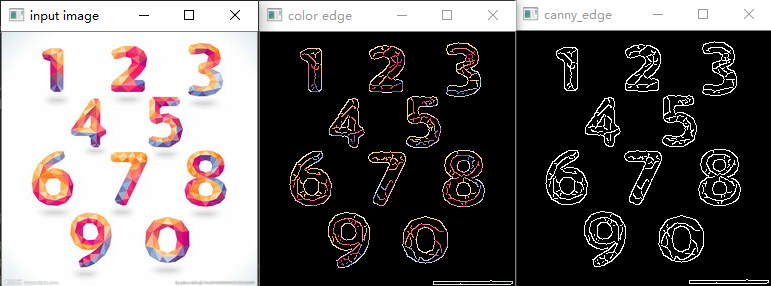opencv
更多相关内容
• Python代码实现Canny算法——图像边缘轮廓提取 一、Canny算法主要思路： 1.通过高斯核进行滤波，去除噪声点，使图像平滑 2.通过求取偏导，使用梯度强度和方向来提取边缘轮廓 3.采用非极大值抑制的方法，将提取到的...

# Python代码实现Canny算法——图像边缘轮廓提取

## 一、Canny算法主要思路：

1.通过高斯核进行滤波，去除噪声点，使图像平滑

2.通过求取偏导，使用梯度强度和方向来提取边缘轮廓

3.采用非极大值抑制的方法，将提取到的粗犷的边缘轮廓细化

4.采用双阈值来进一步过滤噪声即过滤掉不想要的线条，同时保证轮廓的连续性

### 1.高斯核滤波

众所周知，正态分布又叫做高斯分布，高斯核滤波其实就是正态分布的一个应用。

高斯分布：(和标准的二维正态分布有点差别)
G σ = 1 / ( 2 π σ 1 σ 2 ) ∗ e − ( x − μ ) 2 / σ 1 2 + ( y − μ ) 2 ) / σ 2 2 G_σ=1/(2πσ_1σ_2)*e^{-(x-μ)^2/σ_1^2+(y-μ)^2)/σ_2^2}
我们假设一个3*3的高斯核：（我用Excel画的）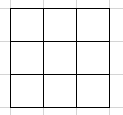设高斯核中小格子的坐标为(x,y)， 高斯核中间的小格子为（0,0），左上角的小格子为（-1，-1），以此类推。

将高斯核中每个小格子的坐标带入到高斯分布公式中，就可以得到每个小格子的值。

高斯核中的所有值得核应该为1，因此需要进行归一化处理，即求出每个小格子占所有小格子总和的权重。

#### 高斯核的应用——卷积

图像是以像素值来表示的。

如图为一个36*36的灰度图像，每个小格子中都有一个像素值，将高斯核覆盖到图像上，如图红色和黑色格子。计算图像和高斯核对应格子的值，并求其总和，即为黑色格子进行高斯卷积之后所对应的像素值。理论上计算时，通常会对高斯核进行上下镜像，之后再进行计算，但实际应用中通常不考虑该镜像操作。

以此类推，可计算整个图像中所有格子进行卷积后的值。再将这些值输出，即可得到进行高斯卷积之后的图像。

高斯卷积会使得图像丢失最外围的像素。因此通常会在卷积之前，在图像的周围填充一些像素，来保证图像卷积后大小不变。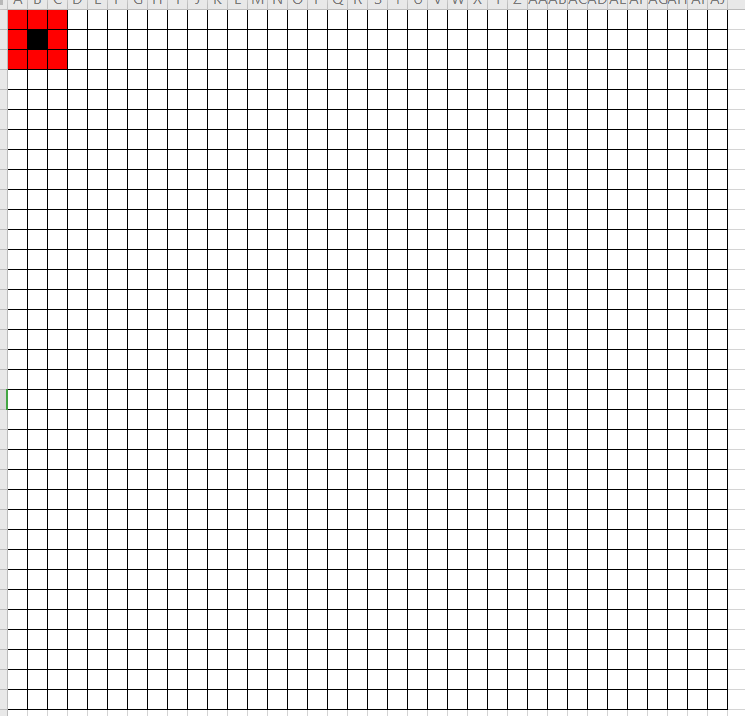### 2.偏导和梯度

为什么要求偏导或者梯度？

图像的边缘是由于像素值的突变引起的，因此我们需要通过求偏导或者梯度来获取到突变值发生的位置，提取轮廓。

（公式不好打，上图吧)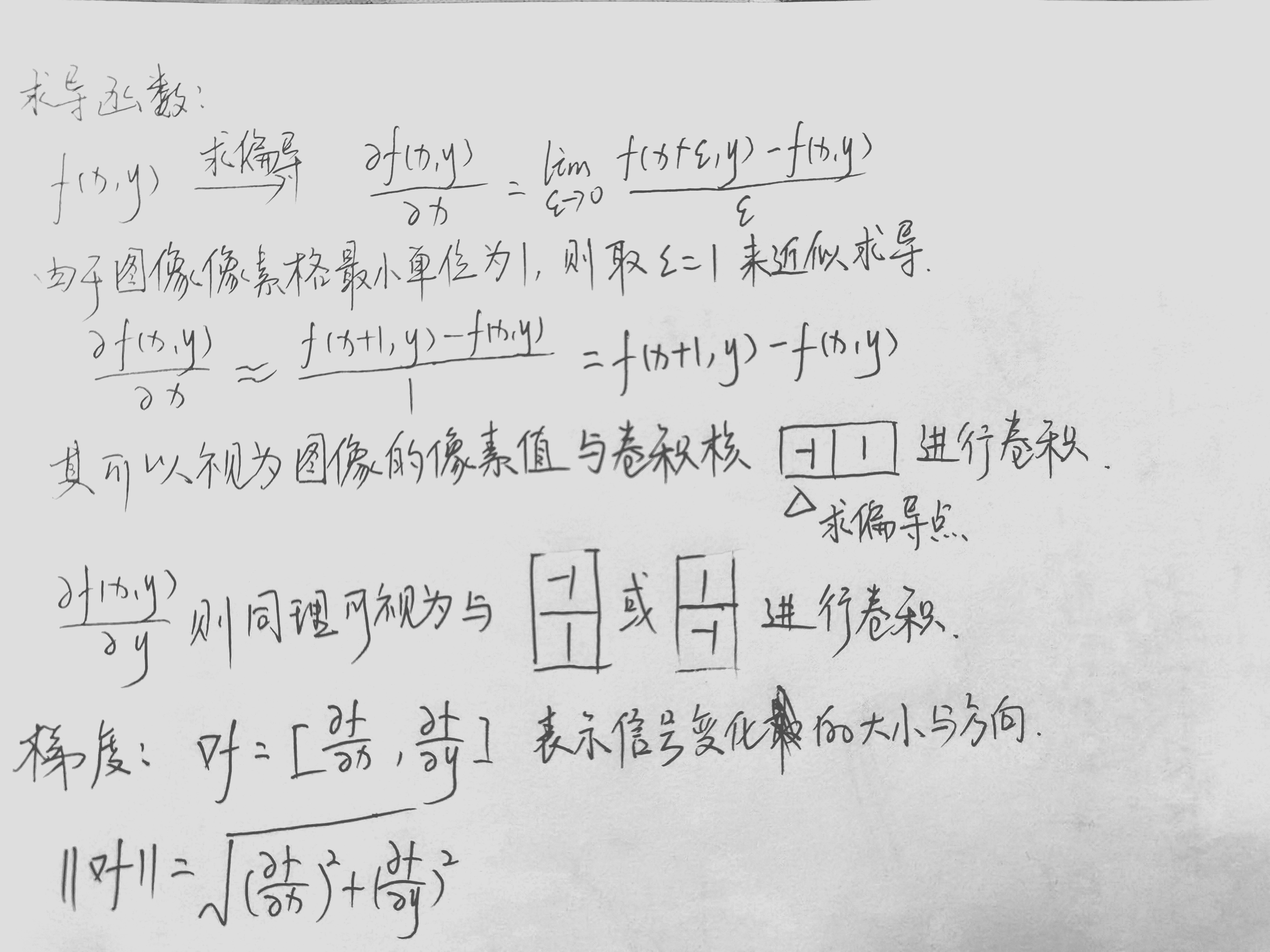求偏导或者梯度函数，其本质上还是和高斯卷积是类似的。

常用的几个求偏导和梯度的模板：

Prewitt模板算子：
M x = [ − 1 0 1 − 1 0 1 − 1 0 1 ] M y = [ 1 1 1 0 0 0 − 1 − 1 − 1 ] M_x = \left[ \begin{array}{ccccc} -1 & 0 & 1\\ -1 & 0 & 1\\ -1 & 0 & 1 \end{array} \right] M_y = \left[ \begin{array}{ccccc} 1 & 1 & 1\\ 0 & 0 & 0\\ -1 & -1 & -1 \end{array} \right]
Sobel模板算子：
M x = [ − 1 0 1 − 2 0 2 − 1 0 1 ] M y = [ 1 2 1 0 0 0 − 1 − 2 − 1 ] M_x = \left[ \begin{array}{ccccc} -1 & 0 & 1\\ -2 & 0 & 2\\ -1 & 0 & 1 \end{array} \right] M_y = \left[ \begin{array}{ccccc} 1 & 2 & 1\\ 0 & 0 & 0\\ -1 & -2 & -1 \end{array} \right]

### 3.非极大值抑制法

由于图像的像素是逐渐进行过渡的，因此我们通过上述过程提取到的边缘会很粗，我们想要得到的图像的边缘应该只有一个像素的粗细。因此我们要提取突变最大的地方作为图像的边缘。

非极大值抑制法，即沿梯度方向，选取梯度强度最大的一个像素块进行保留，将其余像素块舍弃的方法。

比较方法通常为：沿梯度方向，取一像素点，和该像素点前后各一像素的像素点，进行比较，保留最大值。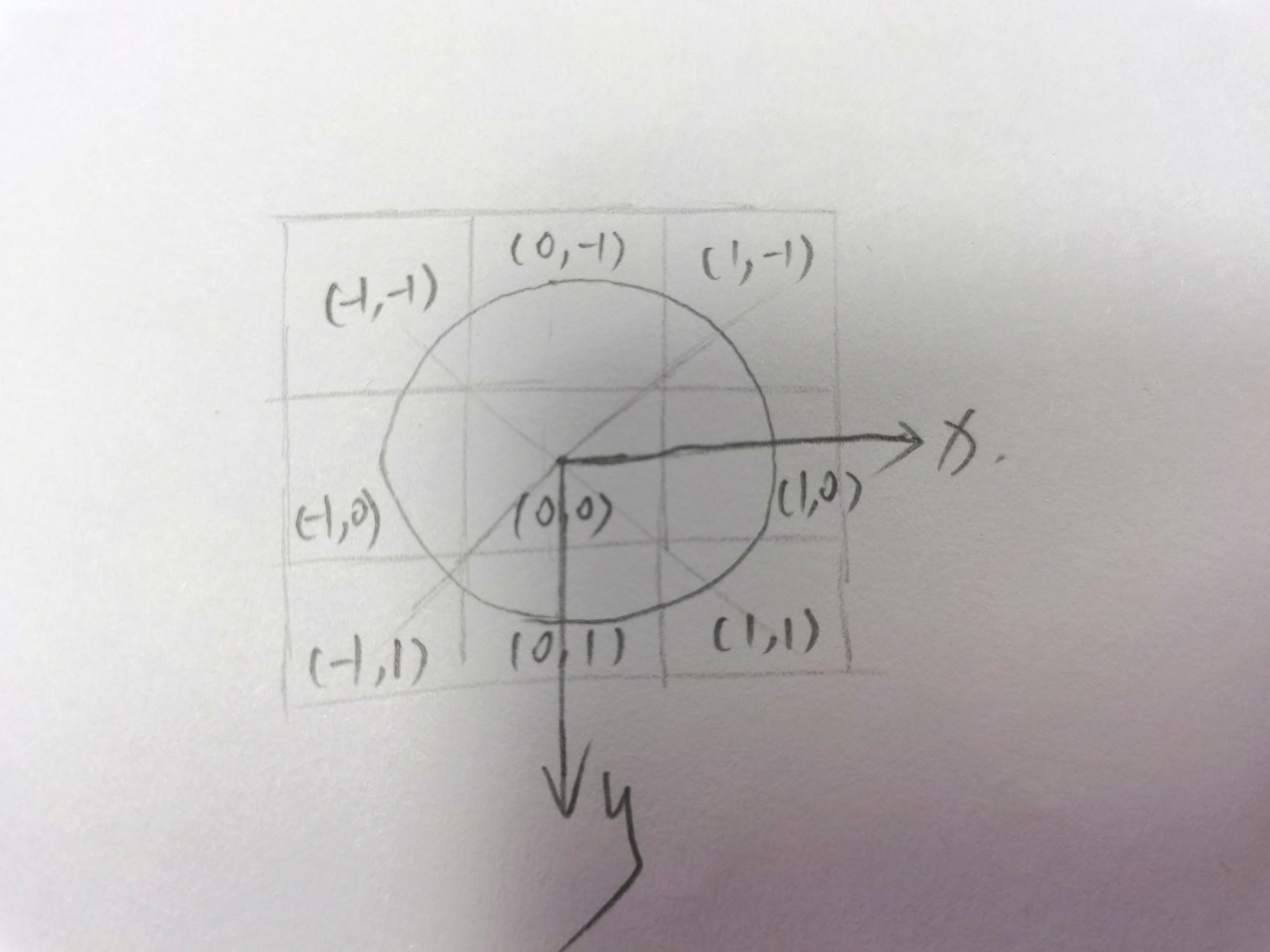如图，假设该3*3的核的每一个格子均在所提出的轮廓线上。

假设梯度方向是沿x轴方向的，我们需要比较点（-1，0）和点（0，0）两处的梯度强度，若点（0，0）的梯度强度大于（-1，0），则比较（0,0）和（1,0）的梯度强度，若（0,0）小于（1,0），则继续比较（1,0）和（2,0），若（0,0）大于（-1,0）且大于（1,0）则（0,0）最大，可保留，其余的均丢弃，即像素值设为0.

然而实际应用中，梯度方向和边垂直，并不都是水平或者垂直的，因此我们可以选取其所靠近的值，采用不同的权重来计算该点的梯度强度。

如下图。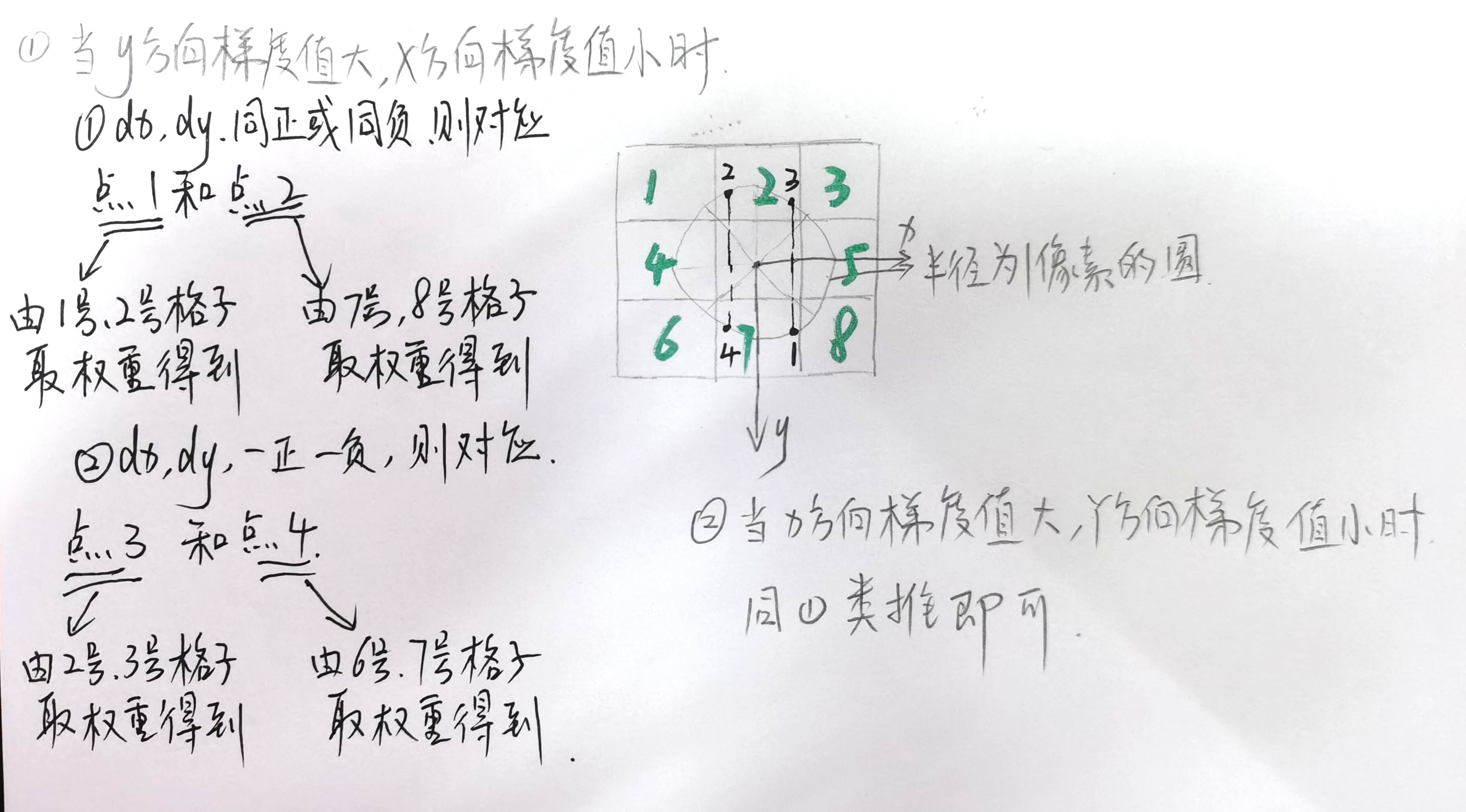### 4.双阈值滤波

双阈值滤波是基于如下的假设进行的：

假设，所有噪声所产生的线条与图像的轮廓是不发生连接的。

这样，我们就可以设置两个阈值，一个高阈值，一个低阈值。低阈值用来过滤噪声和突变不明显的线条，高阈值用来保留轮廓线。在低阈值和高阈值之间，且和轮廓线相邻的线条可保留，否则舍弃。

## 二、代码实现

（代码仅供学习参考使用，尚有不足和待优化之处）

import math
import numpy as np
import matplotlib.pyplot as plt

# 生成高斯核
def gaussian_create():
sigma1 = sigma2 = 1
gaussian_sum = 0
g = np.zeros([3, 3])
for i in range(3):
for j in range(3):
g[i, j] = math.exp(-1 / 2 * (np.square(i - 1) / np.square(sigma1)
+ (np.square(j - 1) / np.square(sigma2)))) / (
2 * math.pi * sigma1 * sigma2)
gaussian_sum = gaussian_sum + g[i, j]
g = g / gaussian_sum  # 归一化
return g

# 产生灰度图
def gray_fuc(rgb):
return np.dot(rgb[..., :3], [0.299, 0.587, 0.114])

# 高斯卷积
def gaussian_blur(gray_img, g):
'''
gray_img:灰度图
g:高斯核
'''
gray_img = np.pad(gray_img, ((1, 1), (1, 1)), constant_values=0)  # 填充
h, w = gray_img.shape
new_gray_img = np.zeros([h - 2, w - 2])
for i in range(h - 2):
for j in range(w - 2):
new_gray_img[i, j] = np.sum(gray_img[i:i + 3, j:j + 3] * g)
return new_gray_img

# 求高斯偏导
def partial_derivative(new_gray_img):
'''
new_gray_img:高斯卷积后的灰度图
'''
new_gray_img = np.pad(new_gray_img, ((0, 1), (0, 1)), constant_values=0)  # 填充
h, w = new_gray_img.shape
dx_gray = np.zeros([h - 1, w - 1])  # 用来存储x方向偏导
dy_gray = np.zeros([h - 1, w - 1])  # 用来存储y方向偏导
df_gray = np.zeros([h - 1, w - 1])  # 用来存储梯度强度
for i in range(h - 1):
for j in range(w - 1):
dx_gray[i, j] = new_gray_img[i, j + 1] - new_gray_img[i, j]
dy_gray[i, j] = new_gray_img[i + 1, j] - new_gray_img[i, j]
df_gray[i, j] = np.sqrt(np.square(dx_gray[i, j]) + np.square(dy_gray[i, j]))
return dx_gray, dy_gray, df_gray

# 非极大值抑制
def non_maximum_suppression(dx_gray, dy_gray, df_gray):
'''
dx_gray:x方向梯度矩阵
dy_gray:y方向梯度矩阵
df_gray:梯度强度矩阵
'''
df_gray = np.pad(df_gray, ((1, 1), (1, 1)), constant_values=0)  # 填充
h, w = df_gray.shape
for i in range(1, h - 1):
for j in range(1, w - 1):
if df_gray[i, j] != 0:
gx = math.fabs(dx_gray[i - 1, j - 1])
gy = math.fabs(dy_gray[i - 1, j - 1])
if gx > gy:
weight = gy / gx
grad1 = df_gray[i + 1, j]
grad2 = df_gray[i - 1, j]
if gx * gy > 0:
grad3 = df_gray[i + 1, j + 1]
grad4 = df_gray[i - 1, j - 1]
else:
grad3 = df_gray[i + 1, j - 1]
grad4 = df_gray[i - 1, j + 1]
else:
weight = gx / gy
grad1 = df_gray[i, j + 1]
grad2 = df_gray[i, j - 1]
if gx * gy > 0:
grad3 = df_gray[i + 1, j + 1]
grad4 = df_gray[i - 1, j - 1]
else:
grad3 = df_gray[i + 1, j - 1]
grad4 = df_gray[i - 1, j + 1]
if df_gray[i, j] > t1 and df_gray[i, j] > t2:
df_gray[i, j] = df_gray[i, j]
else:
df_gray[i, j] = 0
return df_gray

# 双阈值过滤
def double_threshold(df_gray, low, high):
'''
df_gray:梯度强度矩阵
low:低阈值
high:高阈值
'''
h, w = df_gray.shape
for i in range(1, h - 1):
for j in range(1, w - 1):
if df_gray[i, j] < low:
df_gray[i, j] = 0
elif df_gray[i, j] > high:
df_gray[i, j] = 1
elif (df_gray[i, j - 1] > high) or (df_gray[i - 1, j - 1] > high) or (
df_gray[i + 1, j - 1] > high) or (df_gray[i - 1, j] > high) or (df_gray[i + 1, j] > high) or (
df_gray[i - 1, j + 1] > high) or (df_gray[i, j + 1] > high) or (df_gray[i + 1, j + 1] > high):
df_gray[i, j] = 1
else:
df_gray[i, j] = 0
return df_gray

if __name__ == '__main__':
# 读取图像
# 生成高斯核
gaussian = gaussian_create()
# 生成灰度图
gray = gray_fuc(img)
# 高斯卷积
new_gray = gaussian_blur(gray, gaussian)
# 求偏导
d = partial_derivative(new_gray)
dx = d
dy = d
df = d
# 非极大值抑制
new_df = non_maximum_suppression(dx, dy, df)
# 双阈值过滤,并将图像转换成转化二值图
low_threshold = 0.15 * np.max(new_df)
high_threshold = 0.2 * np.max(new_df)
result = double_threshold(new_df, low_threshold, high_threshold)
# 输出图像
plt.imshow(result, cmap="gray")
plt.axis("off")
plt.show()


### 运行结果

（不晓得为啥上次上传的图片显示违规。。。换了张图）上图为原图，下图为提取到的轮廓。运行时间大约44s更换图片，则要根据需要调整双阈值滤波的高低阈值大小，来获得满意的输出。

### 代码改进

上述代码中采用的双阈值过滤函数，是判断弱边周围是否存在强边，来进而确定该弱边是否是我们所需要的边，是否进行滤除。该方法的缺陷：

强边为H，其上有一点a与弱边相连。该弱边为L，其上有一点b和一点c，b点和a点相连。
如果，在判断弱边是否滤除的时候，先判断b点，后判断c点，得知b点和a点相连，b点设为1值保留，c点和b点相连，因此c点也设为1值保留
如果，先判断c点，后判断b点，那么得出的结论是：c点周围没有1值，丢弃。这将导致我们丢失掉我们想要的点

因此改变双阈值过滤函数的算法思想，判断强边周围是否存在弱边，即通过强边延伸弱边。这样可以使我们提取到的边更加的完整。

# 双阈值过滤
def double_threshold(dx_gray, dy_gray, df_gray, low, high):
'''
dx_gray:x方向梯度矩阵
dy_gray:y方向梯度矩阵
df_gray:梯度强度矩阵
low:低阈值
high:高阈值
'''
h, w = df_gray.shape
for i in range(1, h - 1):
for j in range(1, w - 1):
if df_gray[i, j] < low:
df_gray[i, j] = 0
elif df_gray[i, j] >= high:
df_gray[i, j] = 1
if dy_gray[i-1, j-1] * dx_gray[i-1, j-1] > 0:  # dx，dy同向
if df_gray[i - 1, j + 1] > low:
df_gray[i - 1, j + 1] = high
if df_gray[i + 1, j - 1] > low:
df_gray[i + 1, j - 1] = high
if dy_gray[i-1, j-1] > dx_gray[i-1, j-1]:
if df_gray[i, j + 1] > low:
df_gray[i, j + 1] = high
if df_gray[i, j - 1] > low:
df_gray[i, j - 1] = high
else:
if df_gray[i - 1, j] > low:
df_gray[i - 1, j] = high
if df_gray[i + 1, j] > low:
df_gray[i + 1, j] = high
else:
if df_gray[i - 1, j - 1] > low:
df_gray[i - 1, j - 1] = high
if df_gray[i + 1, j + 1] > low:
df_gray[i + 1, j + 1] = high
if math.fabs(dy_gray[i-1, j-1]) > math.fabs(dx_gray[i-1, j-1]):
if df_gray[i, j + 1] > low:
df_gray[i, j + 1] = high
if df_gray[i, j - 1] > low:
df_gray[i, j - 1] = high
else:
if df_gray[i - 1, j] > low:
df_gray[i - 1, j] = high
if df_gray[i + 1, j] > low:
df_gray[i + 1, j] = high
else:
df_gray[i, j] = 0
return df_gray


### 优化结果：

优化后，运行时间增加了￣□￣｜｜，为46s，不过值得一提是，提取的轮廓质量更好了。原先想要的但是没能够输出的边，竟然输出了！ 而且花瓣的轮廓提取更加连续了。
如下上图为未优化前提取的轮廓，下图为优化后提取的轮廓。效果还是不错的。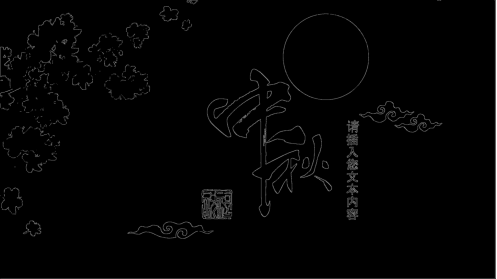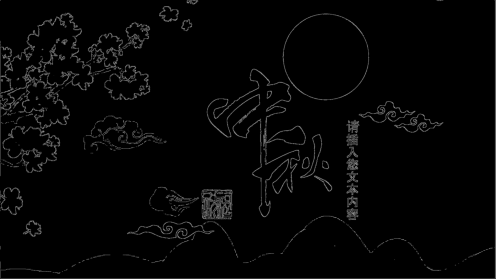### 总结：

基本实现了图像轮廓的提取，但是运行时间较长，代码还需要优化。

## 参考资料：

canny 算子python实现

展开全文计算机视觉
• 在图像处理中，边缘提取是一种基础的图像处理算法，常用于图像识别以及跟踪领域，为进一步分析和理解图像做准备，下面介绍两种不同的图像边缘检测方法。 二、边缘检测方法 本文介绍的边缘检测方法包括直接使用高斯...

## 一、简介

在图像处理中，边缘提取是一种基础的图像处理算法，常用于图像识别以及跟踪领域，为进一步分析和理解图像做准备，下面介绍两种不同的图像边缘检测方法。

## 二、边缘检测方法

本文介绍的边缘检测方法包括直接使用高斯滤波器检测和Canny边缘检测两种方法。

## 1.高斯滤波器提取边缘特征

高斯滤波是一种线性平滑滤波，可以用于消除图像中的高斯噪声。简言之，高斯滤波就是对整幅图像进行加权平均的过程，每一个像素点的值，都由其本身和邻域内的其他像素值经过加权平均后得到。高斯滤波的具体操作是：用一个模板（或称卷积、掩模）扫描图像中的每一个像素，用模板确定的邻域内像素的加权平均灰度值去替代模板中心像素点的值。下面用代码显示一下处理效果：

import cv2
blurred = cv2.GaussianBlur(img,(11,11),0) #高斯矩阵的长与宽都是11，标准差为0
gaussImg = img - blurred
cv2.imshow("Image",gaussImg)
cv2.waitKey(0)


原图：处理后：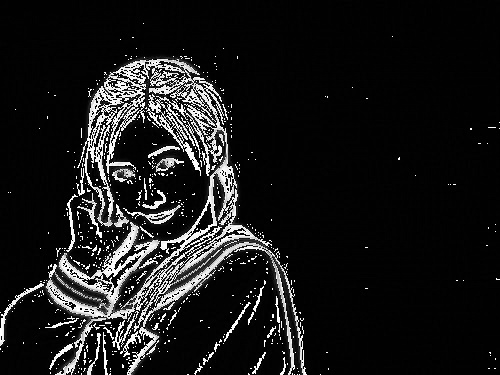## 2.Canny边缘特征提取

Canny边缘检测是一种非常流行的边缘检测算法，是由John F.Canny 在1986 年提出的，Canny边缘检测算法被很多人推崇为当今最优秀的边缘检测算法，关键步骤有两步，即图像去噪和设置阈值来确定边界。
图像去噪：由于边缘检测很容易受到噪声的影响，所以首先要使用高斯滤波器来去除噪声；
设置阈值：对于边界的确定需要我们来设置两个阈值，minv和maxv，当图像的灰度梯度高于maxv时，被认定为真的边界，图像的灰度小于minv时，则被舍弃，倘若灰度梯度介于两者之间，要看这个点是否与某个被认定为真的边界点相连，如果是，就被认为是真的边界点，反之则舍弃。如下图所示，A点高于阈值maxv，所以直接被认定真的边界点，B点和C点虽然都介于minv和maxv之间，但是C点与A点相连，所以也被看作是边界点，B点不与边界点相连，所以被舍弃。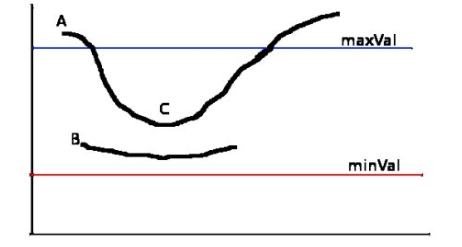下面是Canny边缘检测代码：

import cv2
blurred = cv2.GaussianBlur(img,(11,11),0)
gaussImg = cv2.Canny(blurred, 10, 70)
cv2.imshow("Img",gaussImg)
cv2.waitKey(0)


原图：处理后：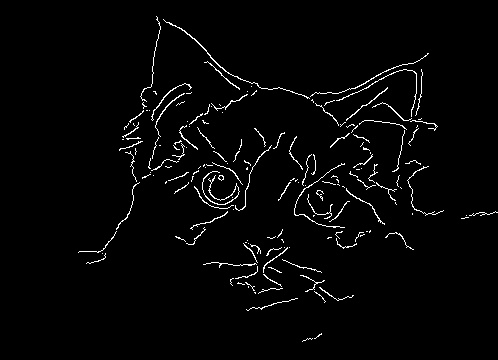以上就是文章的所有内容，如果本文对你有帮助，可以给我点个赞，加个关注，谢谢！

展开全文opencv 计算机视觉
• 实现思路： 　1，将传进来的图片矩阵用算子进行卷积求和（卷积和取绝对值） 　2，用新的矩阵（与原图一样大小）去接收每次的卷积和的值 　3，卷积图片所有的像素点后，把新的矩阵数据类型转化为uint8 ...
• title: "Python实现图像边缘检测算法"date: 2018-06-12T17:06:53+08:00tags: ["图形学"]categories: ["python"]实现效果代码#!/usr/bin/env python3# coding=utf-8from PIL import Imageimport numpy as npimg_name ...

title: "Python实现图像边缘检测算法"

date: 2018-06-12T17:06:53+08:00

tags: ["图形学"]

categories: ["python"]

实现效果代码

#!/usr/bin/env python3

# coding=utf-8

from PIL import Image

import numpy as np

img_name = input("输入要处理的图片\n")

# img_name = "t3.png"

img = Image.open(img_name).convert("L") # 读图片并转化为灰度图

img.show()

img_array = np.array(img) # 转化为数组

w, h = img_array.shape

img_border = np.zeros((w-1, h-1))

for x in range(1, w - 1):

for y in range(1, h - 1):

Sx = img_array[x + 1][y - 1] + 2 * img_array[x + 1][y] + img_array[x + 1][y + 1] - \

img_array[x - 1][y - 1] - 2 * \

img_array[x - 1][y] - img_array[x - 1][y + 1]

Sy = img_array[x - 1][y + 1] + 2 * img_array[x][y + 1] + img_array[x + 1][y + 1] - \

img_array[x - 1][y - 1] - 2 * \

img_array[x][y - 1] - img_array[x + 1][y - 1]

img_border[x][y] = (Sx * Sx + Sy * Sy) ** 0.5

img2 = Image.fromarray(img_border)

img2.show()

展开全文• 今天小编就为大家分享一篇关于Python 的简单栅格图像边界提取方法，具有很好的参考价值，希望对大家有所帮助。一起跟随小编过来看看吧
• ## Python opencv边缘提取

千次阅读 2021-03-11 14:07:10
利用opencv或其他工具编写程序实现图像边缘提取。 实现过程 import cv2 import numpy as np # imread()两个参数： # 1、图片路径。 # 2、读取图片的形式（1：默认值，加载彩色图片。 0：加载灰度图片。 -1：加载...opencv 计算机视觉
• opencv图像处理基础总结，图像金字塔实现对图像的采样；图像梯度(Sobel算子、Scharr算子和拉普拉斯算子)；Canny算法对图像进行边缘检测。opencv Canny算法
• 首先使用canny或其他方法将图片边缘轮廓提取： （下图为mask处理后的图像） 参考论文 《A Sub-Pixel Edge Detector: an Implementation of theCanny/Devernay Algorithm》 提取后的亚像素级边缘散点如下： 由于亚...c语言 数据结构
• plt.imshow(sobel_img_my_cv) plt.title("Using Sobel Lib from Mine,Load cv") plt.show() 运行效果： 红色箭头的所指的图像边缘提取效果比黄色箭头所指向的图像边缘提取效果好。 原因： skimage 加载出来的图像...
• python opencv Sobel、Laplace、canny算子的边缘提取 以及参数解析 前提:各种算子不完全区分好坏,但根据我实际操作分析得到,有的算子之间效果大相径庭,但有的也很相似,也就是各有各的用法,这里按 Sobel.Laplace....
• ④ 根据边缘角度对边缘强度进行非极大值抑制（Non-maximum suppression），使图像边缘变得更细  非极大值抑制算法：0°时取(x,y)、(x+1,y)、(x-1,y) 中的最大值，其它角度类似 ↑ ⑤ 使用滞后阈值对图像
• 本文实例讲述了Python实现使用卷积提取图片轮廓功能。分享给大家供大家参考，具体如下：一、实例描述将彩色的图片生成带边缘化信息的图片。本例中先载入一个图片，然后使用一个“3通道输入，1通道输出的3*3卷积核”...
• 主要介绍了python用opencv完成图像分割并进行目标物的提取，文中通过示例代码介绍的非常详细，对大家的学习或者工作具有一定的参考学习价值，需要的朋友们下面随着小编来一起学习学习吧
• 如果需要检测到图像里面的边缘，首先我们需要知道边缘处具有什么特征。对于一幅灰度图像来说，边缘两边的灰度值肯定不相同，这样我们才能分辨出哪里是边缘，哪里不是。因此，如果我们需要检测一个灰度图像边缘，...
• 但是让计算机去区分这些图片分别是哪一类是很不容易的，不过计算机可以知道图像的像素值的，因此，在图像识别过程中，通过颜色特征来识别是相似图片是我们常用的（当然还有其特征还有纹理特征、...
• 实现snake分割 通过snake方法 对图像边缘进行提取
• 边缘检测是图像处理和计算机视觉的基本问题，边缘检测的目的是标识数字图像中亮度...图像边缘检测大幅度的减少了数据量，并且剔除了可以认为不相关的信息，保留了图像重要的结构属性。在图像中，边缘可以看做是位于...
• 轮廓发现的原理：先通过阈值分割提取目标物体，再通过边缘检测提取目标物体轮廓。 一个轮廓就是一系列的点（像素），这些点构成了一个有序的点集合。 使用 cv2.findContours 函数可以用来检测图像边缘。 函数原型...opencv 计算机视觉 人工智能 机器学习
• 提取图片边缘特征 图像聚类 通过像素点对图片进行聚类图片二值化，转化为黑白图片
• 1.图像二值化 全局阈值分割 “cv.THRESH_TRIANGLE” 只有单个波峰时效果较好，分割细胞图 一般用"cv.THRESH_OTSU" “cv.THRESH_TRUNC”,截断，大于阈值设为阈值 “THRESH_TOZERO”,小于阈值设为0 cv.threshold...opencv
• 图像边缘检测大幅度地减少了数据量，并且剔除了可以认为不相关的信息，保留了图像重要的结构属性。有许多方法用于边缘检测，它们的绝大部分可以划分为两类：基于查找一类和基于零穿越的一类。基于查找的方法通过寻找...
• 这篇文章主要介绍了opencv python Canny边缘提取实现过程解析,文中通过示例代码介绍的非常详细，对大家的学习或者工作具有一定的参考学习价值,需要的朋友可以参考下Canny是边缘提取算法，在1986年提出的是一个很好的...
• (1)最优检测：算法能够尽可能多地标识出图像中的实际边缘，漏检真实边缘的概率和误检非边缘的概率都尽可能小； (2)最优定位准则：检测到的边缘点的位置距离实际边缘点的位置最近，或者是由于噪声影响引起检测出的...算法 计算机视觉
• ## Python图片边缘匹配

千次阅读 2022-01-04 15:50:18
def get_gap(image1,image2,bg_filenam,sd_filenam): #读取图片 ... #识别图片边缘 bg_edge = cv2.Canny(bg_image,20,100) sd_edge = cv2.Canny(slider_img,20,100) #转换图片格式 bg_pic = cv2.c计算机视觉
• 去除噪声由于边缘检测容易受到图像中噪声的影响3.找寻图像的强度梯度Canny的基本思想是找寻一幅图像中强度变化最强的位置。所谓的变化最强，即指梯度方向。平滑后的图像中每个像素点的梯度可以由S...
• 实现图像边缘提取算法，采用robert算子、prewitt算子、sobel算子对图像进行边缘提取。matlab实现。
• DoH方法就是利用图像点二阶微分Hessian矩阵, Hessian矩阵行列式的值，同样也反映了图像局部的结构信息。与LoG相比，DoH对图像中的细长结构的斑点有较好的抑制作用。无论是LoG还是DoH，它们对图像中的斑点进行检测，......